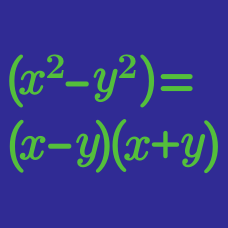Algebra

# Factoring Cubic Polynomials

What real value of $x$ satisfies the equation $\frac{x^3-21x^2+20x}{x-1}= -100?$

The cubic equation $x^3-7x^2-x+7=0$ has three real roots. Let $A$ be the greatest root and $B$ be the least root. What is the value of $A-B$?

Given $2x^3+17x^2+19x-14=(2x-a)(x+b)(x+c),$ what is the value of $a+b+c$?

The polynomial in two variables

$P(x,y) = x^3 - 10 y^2 + 10 x^2 - y^3$

can be expressed as

$P(x,y) = (ax+by+c)(dx^2+exy + fy^2 + gx + hy + i ),$

where the variables from $a$ to $i$ are all integers. What is the value of $| d + e + f + g + h + i |$?

If $a,b,$ and $c$ are positive numbers such that $x^3-6x^2-37x-30=(x+a)(x+b)(x-c),$ what is the value of $a+b+c$?

×